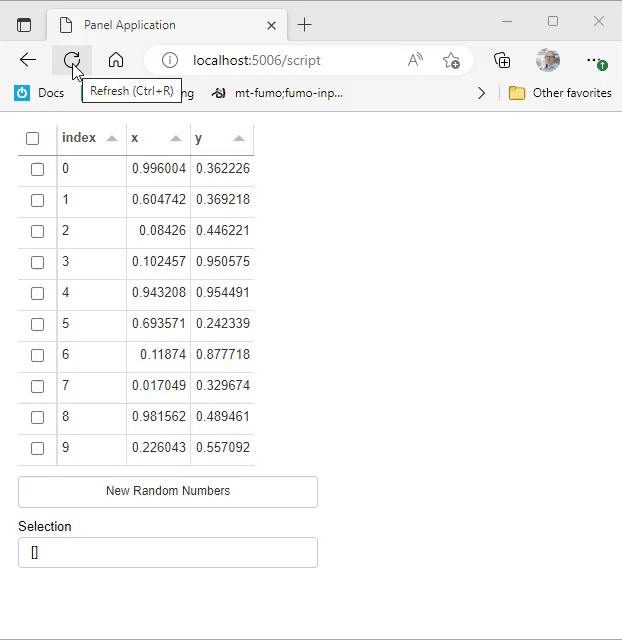# How to access params of a Tabulator that is dynamically updated

I have a dynamically updated tabulator, and I don’t know how to access its selected rows. Hope the example is understandable.

``````import param
import panel as pn
import pandas as pd
import numpy as np
pn.extension("tabulator")

class DataProfile(param.Parameterized):

_df = param.DataFrame(doc="Values as Pandas DataFrame")

button = param.Event(label='new random numbers')

def __init__(self, **params):
super().__init__(**params)

def table(self):
table_ = pn.widgets.Tabulator(self._df, selectable='checkbox')
pn.bind(table_.param.values, self._df)
return table_

@pn.depends("button", watch=True)
def _update_dataframe(self):
self._df = pd.DataFrame(dict(zip("xy",np.random.rand(2,10))))

app = DataProfile(
_df=pd.DataFrame({
"x": [0, 5, 10],
"y": [0, 3, 10]
}),
)

tbl = pn.panel(app.table)
layout = pn.Column(
tbl,
app.param.button,
### none of these work
# tbl._pane.selection,
# tbl._pane.param.selection,
# tbl.selection,
)
layout.servable()
``````

Ideally, I would like to have inside the class.

If you find some other suggestions to the code, please feel free to comment too.

1 Like

Hi @szarma

If you include `tbl.param.selection` instead of `tbl`selection`in the`Column` it should work for you.

This refactored example works for me.

``````import param
import panel as pn
import pandas as pd
import numpy as np
pn.extension("tabulator")

def get_random_data():
return pd.DataFrame(dict(zip("xy",np.random.rand(2,10))))

class DataProfile(param.Parameterized):

data = param.DataFrame(doc="Values as Pandas DataFrame")
update_data = param.Event(label='New Random Numbers')

def __init__(self, **params):
super().__init__(**params)

def create_table(self):
table_ = pn.widgets.Tabulator.from_param(self.param.data, selectable='checkbox')
return table_

@pn.depends("update_data", watch=True)
def _update_dataframe(self):
self.data = get_random_data()

app = DataProfile(data=get_random_data())

tbl = app.create_table()
layout = pn.Column(
tbl,
app.param.update_data,
tbl.param.selection,
)
layout.servable()
``````Thanks, @Marc. This does work in deed.

Do you also happen to know is there a way to have the `Tabulator` inside the app somehow, as a parameter? I have the feeling it would offer a more natural interaction between the objects.

The way it is now, I need to go “outside” of the scope of the class, and then “back inside” to use its methods.

Let me illustrate

``````import param
import panel as pn
import pandas as pd
import numpy as np
import holoviews as hv
hv.extension('bokeh')
pn.extension("tabulator")

def get_random_data():
return pd.DataFrame(dict(zip("xy",np.random.rand(2,10))))

class DataProfile(param.Parameterized):

data = param.DataFrame(doc="Values as Pandas DataFrame")
update_data = param.Event(label='New Random Numbers')

def __init__(self, **params):
super().__init__(**params)

def create_table(self):
table_ = pn.widgets.Tabulator.from_param(self.param.data, selectable='checkbox')
return table_

@pn.depends("update_data", watch=True)
def _update_dataframe(self):
self.data = get_random_data()

############# the only different part #############
@pn.depends("data")
def plot_selected(self,selection=None):
# this is a method that I would like to use
if selection is None or len(selection)==0:
selection = self.data.index
else:
selection = list(selection)
return hv.Points(self.data.loc[selection])

app = DataProfile(data=get_random_data())

# here I create the Tabulator outside of the DataProfile scope
tbl = app.create_table()
layout = pn.Column(
tbl,
app.param.update_data,
# here I'm back to using the DataProfiler method
hv.DynamicMap(app.plot_selected, streams=[tbl.param.selection]),
)
layout.servable()
``````

This is how my code looks like currently, but I feel like the holoviz developers have provided all these awesome and elegant solutions and bindings, that I am certain I am just missing something when I find myself needing to reach for what feels like a hacky implementation.

All in all, I am probably overthinking thisI have the feeling Marc’s suggestion is close to the best you can get. It is true that `panel` can map `param` Parameters (Number, String, Boolean, Selector, etc.) to widgets and thanks to that you can evolve in `param`'s world to code your apps. Representing in a GUI interface like `panel` one of these simpler Parameters (e.g. a Number into a FloatSlider) is rather straightforward. But all the ways you can interact with a Dataframe mean that it would certainly not make sense to change `param` to support them, `param` isn’t only about GUI programming after all. So in these cases you have to go into `panel`'s world more. I use `from_param` quite a lot to do that, I find it a natural pattern to keep things in sync.

3 Likes

Thanks for providing the context. And for pointing out the `from_param` method. I somehow missed it while going through tutorials.

1 Like